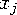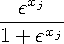Functions and CALL Routines

# CALL LOGISTIC Routine

Applies the logistic function to each argument.
 Category: Mathematical

## Syntax

 CALL LOGISTIC(argument<, argument, ...>)

### Arguments

argument

is a numeric variable.

 Restriction The CALL LOGISTIC routine only accepts variables as valid arguments. Do not use a constant or a SAS expression because the CALL routine is unable to update these arguments.

The CALL LOGISTIC routine replaces each argument by the logistic value of that argument. For exampleis replaced byIf any argument contains a missing value, then CALL LOGISTIC returns missing values for all the arguments.

The following SAS statements produce these results.

SAS Statements Results
```x=0.5;
y=-0.5;
call logistic(x,y);
put x= y=;```
```

x=0.6224593312 y=0.3775406688```Previous Page | Next Page | Top of Page